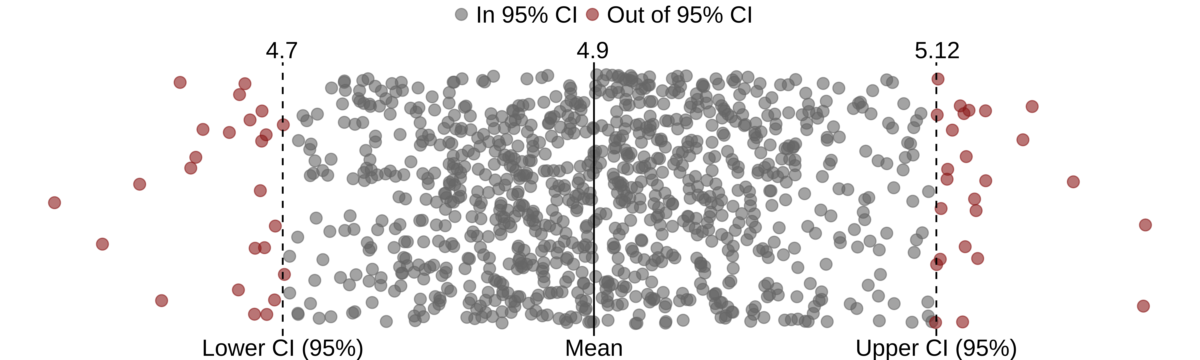# Rsample (Part 1) – Bootstrap estimate of a confidence interval for a mean

The Rsample package contains functions that allow different types of resampling (e.g. cross-validation, bootstrap, etc.). The data structure in which resampling data is stored is a data frame and is very convenient for further work. You can read more about the Rsample package on the official package page: https://github.com/tidymodels/rsample. The package is part of the RStudio “tidymodels” project (project leader is Max Kuhn).

In the first post about the Rsample package, we will show its application for estimating confidence interval of the mean. This example will show the basic structure of the package. We can present the task in the following way. We want to estimate the 95% confidence interval of the mean, and we do not know the analytical apparatus for evaluating the interval, in this case the t-test. The idea is to create a large number of bootstrap samples (repeat samples of the same size as the original sample) from the original sample and to calculate the mean value for each. The estimate of the confidence interval would then be calculated by simply calculating the 0.025 and 0.975 quantiles from the obtained mean values.

First, we will create a sample from a normal distribution with a mean value of 5 and standard deviation 1. The sample size is 100.

```library(rsample)
library(ggplot2)
library(dplyr)
library(purrr)

set.seed(54321)
data <- data_frame(x = rnorm(100, 5))
data
```
```# A tibble: 100 x 1
x
<dbl>
1  4.82
2  4.07
3  4.22
4  3.35
5  4.59
6  3.90
7  3.31
8  7.52
9  6.40
10  5.18
# ... with 90 more rows
```

We will use the bootstraps function from the Rsample package to generate 1000 bootstrap samples. Generated samples are stored in the data frame in the splits column. Column id denotes the name of each sample.

```bt_data <- bootstraps(data, times = 1000)
bt_data
```
```# Bootstrap sampling
# A tibble: 1,000 x 2
splits       id
<list>       <chr>
1 <S3: rsplit> Bootstrap0001
2 <S3: rsplit> Bootstrap0002
3 <S3: rsplit> Bootstrap0003
4 <S3: rsplit> Bootstrap0004
5 <S3: rsplit> Bootstrap0005
6 <S3: rsplit> Bootstrap0006
7 <S3: rsplit> Bootstrap0007
8 <S3: rsplit> Bootstrap0008
9 <S3: rsplit> Bootstrap0009
10 <S3: rsplit> Bootstrap0010
# ... with 990 more rows
```

The structure of the first bootstrap sample can be seen via the following command:

```bt_data\$splits[]
```
```<100/39/100>
```

We see that the new sample contains 100 observations (analysis data in the terminology of the Rsample package), 39 observations were in the assessment (often used to evaluate the performance of a model that was fit to the analysis data). The last number indicates the size of the original set. This data is expected as it is a sample with replacement. Each new sample can be accessed using the analysis(sample) function. Assessment data is accessed using the assessment(sample) function.

Once we have created bootstrap samples the mean value for each sample is calculated. We create an auxiliary function get_split_mean to calculate the sample mean value and later apply it to each sample.

```get_split_mean <- function(split) {
split_data <- analysis(split)
# calculate the sample mean value
split_mean <- mean(split_data\$x)
return(split_mean)
}
```

Using the map_dbl function from the purrr package, we will pass through all the samples and calculate the mean values. We add the new vector of mean values to the existing bt_data data frame. In this way, we get a very nice structure (tidy data) for further work.

```bt_data\$bt_means <- map_dbl(bt_data\$splits, get_split_mean)
bt_data
```
```# Bootstrap sampling
# A tibble: 1,000 x 3
splits       id            bt_means
<list>       <chr>            <dbl>
1 <S3: rsplit> Bootstrap0001     5.02
2 <S3: rsplit> Bootstrap0002     4.93
3 <S3: rsplit> Bootstrap0003     4.99
4 <S3: rsplit> Bootstrap0004     4.86
5 <S3: rsplit> Bootstrap0005     4.90
6 <S3: rsplit> Bootstrap0006     5.03
7 <S3: rsplit> Bootstrap0007     5.10
8 <S3: rsplit> Bootstrap0008     5.00
9 <S3: rsplit> Bootstrap0009     4.78
10 <S3: rsplit> Bootstrap0010     5.07
# ... with 990 more rows
```

The 95% confidence interval is obtained using the quantile function.

```bt_ci <- round(quantile(bt_data\$bt_means, c(0.025, 0.975)), 3)
bt_ci
```
``` 2.5% 97.5%
4.704 5.117
```

We calculate the confidence interval of the original set using the t.test function.

```t_test <- t.test(data\$x)
tt_ci <- round(t_test\$conf.int, 3)
tt_ci
```
``` 4.697 5.117
attr(,"conf.level")
 0.95
```

We see that the intervals are almost identical. In the same way we can estimate other parameters for which an analytical solution can’t be obtained or the solution is too complex.

The estimate of the confidence interval using bootstrap samples is shown in the following graph. The code for the graph is in the Appendix.#### Appendix

```# Uploading required R packages
library(rsample)
library(ggplot2)
library(dplyr)
library(purrr)

# Generating sample from a normal distribution
set.seed(54321)
data <- data_frame(x = rnorm(100, 5))
data

# Generate 1000 bootstrap samples
bt_data <- bootstraps(data, times = 1000)
bt_data

# Display the structure of the first bootstrap sample
bt_data\$splits[]

# Create an auxiliary function for calculating the sample mean value
get_split_mean <- function(split) {
split_data <- analysis(split)
# calculate the sample mean value
split_mean <- mean(split_data\$x)
return(split_mean)
}

# Adding the new vector to the existing data frame.
bt_data\$bt_means <- map_dbl(bt_data\$splits, get_split_mean)
bt_data

# Calculate the 95% confidence interval.
bt_ci <- round(quantile(bt_data\$bt_means, c(0.025, 0.975)), 3)
bt_ci

# Calculate the confidence interval of the original set.
t_test <- t.test(data\$x)
tt_ci <- round(t_test\$conf.int, 3)
tt_ci

#################
# Generate graph
##################
bt_ci_lower <- quantile(bt_data\$bt_means, c(0.025))
bt_ci_upper <- quantile(bt_data\$bt_means, c(0.975))
bt_mean <- mean(bt_data\$bt_means)

bt_data <- bt_data %>%
dplyr::mutate(Color = ifelse(bt_means < bt_ci_lower | bt_means > bt_ci_upper, "Out of 95% CI", "In 95% CI"))

ggplot(bt_data, aes(x = bt_means, y = 0)) +
geom_jitter(aes(color = Color), alpha = 0.6, size = 3, width = 0) +
geom_vline(xintercept = round(c(bt_ci_lower, bt_mean, bt_ci_upper),2), linetype = c(2,1,2)) +
scale_x_continuous(breaks = round(c(bt_ci_lower, bt_mean, bt_ci_upper),2),
labels = c("Lower CI (95%)", "Mean", "Upper CI (95%)"),
sec.axis = sec_axis(~., breaks = round(c(bt_ci_lower, bt_mean, bt_ci_upper),2),
labels = round(c(bt_ci_lower, bt_mean, bt_ci_upper),2))) +
scale_color_manual(values = c("gray40", "firebrick4")) +
theme_void() +
theme(legend.title = element_blank(),
legend.position = "top",
legend.text = element_text(size = 14),
axis.text.x = element_text(size = 14))
```